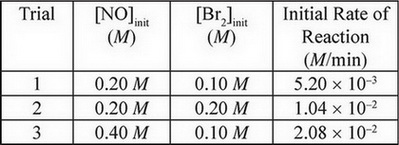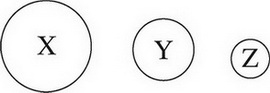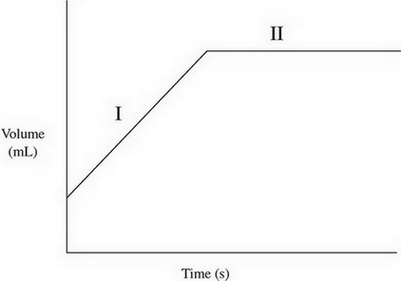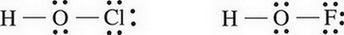1274
Created byMaster Student

Chemistry Practice Test #1

1 / 30

When in liquid state, which two substances are most likely to be miscible with water?

2 / 30

A sample of a compound known to consist of only carbon, hydrogen, and oxygen is found to have a total mass of 29.05 g. If the mass of the carbon is 18.02 g and the mass of the hydrogen is 3.03 g, what is the empirical formula of the compound?

3 / 30

SF4(g) + H2O(1) → SO2(g) + 4HF(g) ΔH = -828 kJ/mol

Which of the following statements accurately describes the above reaction?

4 / 30

During a chemical reaction, NO(g) gets reduced and no nitrogen-containing compound is oxidized. Which of the following is a possible product of this reaction?

5 / 30

2ClF(g) + O2(g) ↔ Cl2O(g) + F2O(g) ΔH = 167 kJ/molrxn

During the reaction above, the product yield can be increased by increasing the temperature of the reaction. Why is this effective?

6 / 30

A compound is made up of entirely silicon and oxygen atoms. If there are 14.00 g of silicon and 32.0 g of oxygen present, what is the empirical formula of the compound?

7 / 30

Which expression below should be used to calculate the mass of copper that can be plated out of a 1.0 M Cu(NO3)2 solution using a current of 0.75 A for 5.0 minutes?

8 / 30

A sealed, rigid container contains three gases: 28.0 g of nitrogen, 40.0 g of argon, and 36.0 g of water vapour. If the total pressure exerted by the gases is 2.0 atm, what is the partial pressure of the nitrogen?

9 / 30

A sample of oxygen gas at 50 °C is heated, reaching a final temperature of 100 °C. Which statement best describes the behaviour of the gas molecules?

10 / 30

Which of the following substances has an asymmetrical molecular structure?

11 / 30

Neutral atoms of chlorine are bombarded by high-energy photons, causing the ejection of electrons from the various filled subshells. Electrons originally from which subshell would have the highest velocity after being ejected?

12 / 30

4NH3(g) + 5O2(g) → 4NO(g) + 6H2O(g)

The above reaction will experience a rate increase by the addition of a catalyst such as platinum. Which of the following best explains why?

13 / 30

2NO(g) + Br2(g) ↔ 2NOBr(g)

The above experiment was performed several times, and the following data was gathered:What is the rate law for this reaction?

14 / 30

The first ionization energy for a neutral atom of chlorine is 1.25 MJ/mol and the first ionization energy for a neutral atom of argon is 1.52 MJ/mol. How would the first ionization energy value for a neutral atom of potassium compare to those value

15 / 30

A sample of liquid NH3 is brought to its boiling point. Which of the following occurs during the boiling process?

16 / 30

The following diagram shows the relative atomic sizes of three different elements from the same period. Which of the following statements must be true?17 / 30

Consider the following reaction showing photosynthesis:

6CO2(g) + 6H2O(l) → C6H12O6(s) + 6O2(g) DH = + 2800 kJ/mol

Which of the following is true regarding the thermal energy in this system?

18 / 30

CH3NH2(aq) + H2O(l) ↔ OH-(aq) + CH3NH3+(aq)

The above equation represents the reaction between the base methylamine (Kb = 4.38 × 10-4) and water. Which of the following best represents the concentrations of the various species at equilibrium?

19 / 30

Between propane and ethene, which will likely have the higher boiling point and why?

20 / 30

2.The volume of a gas is charted over time, giving the above results. Which of the following options provides a possible explanation of what was happening to the gas during each phase of the graph?

21 / 30

For a reaction involving nitrogen monoxide inside a sealed flask, the value for the reaction quotient (Q) was found to be 1.1 × 102 at a given point. If, after this point, the amount of NO gas in the flask increased, which reaction is most likely taking place in the flask?

22 / 30

Starting with a stock solution of 18.0 M H2SO4, what is the proper procedure to create a 1.00 L sample of a 3.0 M solution of H2SO4 in a volumetric flask?

23 / 30

Based on the strength of the intermolecular forces in each substance, estimate from greatest to smallest the vapour pressures of each substance in a liquid state at the same temperature.

24 / 30

Which of the following pairs of substances would make a good buffer solution?

25 / 30

N2(g) + O2(g) + Cl2(g) ↔ 2NOCl(g) Δ = 132.6 kJ/mol

For the equilibrium above, what would happen to the value of Δ if the concentration of N2 were to increase and why?

26 / 30

SO2Cl2 → SO2(g) + Cl2(g)

At 600 K, SO2Cl2 will decompose to form sulfur dioxide and chlorine gas via the above equation. If the reaction is found to be first-order overall, which of the following will cause an increase in the half-life of SO2Cl2?

27 / 30

The structure of two oxoacids is shown below:Which would be a stronger acid, and why?

28 / 30

Nitrogen's electronegativity value is between those of phosphorus and oxygen. Which of the following correctly describes the relationship between the three values?

29 / 30

PCl3(g) + Cl2(g) ↔ PCl5(g) ΔH = -92.5 kJ/mol

In which of the following ways could the reaction above be manipulated to create more product?

30 / 30

A proposed mechanism for a reaction is as follows:

NO2 + F2 →NO2F + F Slow step

F + NO2 → NO2F Fast step

What is the order of the overall reaction?